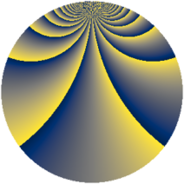# Properties

 Label 2736.2.enLevel $2736$ Weight $2$ Character orbit 2736.en Rep. character $\chi_{2736}(229,\cdot)$ Character field $\Q(\zeta_{12})$ Dimension $1728$ Sturm bound $960$

# Related objects

## Defining parameters

 Level: $$N$$ $$=$$ $$2736 = 2^{4} \cdot 3^{2} \cdot 19$$ Weight: $$k$$ $$=$$ $$2$$ Character orbit: $$[\chi]$$ $$=$$ 2736.en (of order $$12$$ and degree $$4$$) Character conductor: $$\operatorname{cond}(\chi)$$ $$=$$ $$144$$ Character field: $$\Q(\zeta_{12})$$ Sturm bound: $$960$$

## Dimensions

The following table gives the dimensions of various subspaces of $$M_{2}(2736, [\chi])$$.

Total New Old
Modular forms 1936 1728 208
Cusp forms 1904 1728 176
Eisenstein series 32 0 32

## Trace form

 $$1728q + 12q^{6} + O(q^{10})$$ $$1728q + 12q^{6} - 24q^{12} + 20q^{18} + 56q^{24} + 32q^{26} - 24q^{27} - 40q^{30} + 40q^{32} - 52q^{36} + 40q^{42} + 80q^{47} - 52q^{48} + 864q^{49} + 52q^{50} + 40q^{51} - 112q^{54} + 36q^{58} - 24q^{59} - 24q^{60} - 24q^{62} + 72q^{64} - 88q^{66} - 52q^{68} - 60q^{72} - 192q^{78} - 272q^{80} + 72q^{82} + 40q^{83} + 96q^{84} - 144q^{86} + 48q^{88} - 8q^{90} + 76q^{92} + 48q^{93} - 64q^{95} - 16q^{96} - 32q^{99} + O(q^{100})$$

## Decomposition of $$S_{2}^{\mathrm{new}}(2736, [\chi])$$ into newform subspaces

The newforms in this space have not yet been added to the LMFDB.

## Decomposition of $$S_{2}^{\mathrm{old}}(2736, [\chi])$$ into lower level spaces

$$S_{2}^{\mathrm{old}}(2736, [\chi]) \cong$$ $$S_{2}^{\mathrm{new}}(144, [\chi])$$$$^{\oplus 2}$$